##### GED Test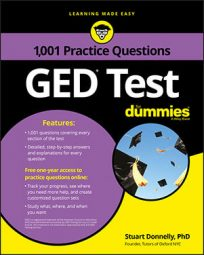Word problems on the GED Math test that involve interest rates are usually straightforward. However, you will need to be familiar with the interest rate formula.

## Practice Questions

1. Arnold invested \$700 in a savings account. After one year, his account balance was \$726.25. What percent annual interest did his bank pay?
2. Amy invested \$500 in a savings account that pays a fixed simple interest rate. After three years, her account balance is \$552.50. What percent annual interest did her bank pay?

A. 3.5% B. 5.5% C. 10.5% D. 17.5%

## Answers and Explanations

1. The correct answer is 3.75%.

You can use the following formula to calculate the annual interest rate: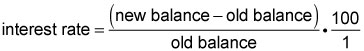Plug in the given values to find the annual interest rate:Hence, the interest rate as 3.75%.

Alternatively, the interest formula is

I = Prt

where I is the interest earned, P is the principal, r is the interest rate, and t is the time. Substituting in the values gives you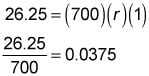To convert this decimal answer into a percentage, simply multiply it by 100 to get 3.75%.

2. The correct answer is 3.5%.

You can use the following formula to calculate the annual interest rate: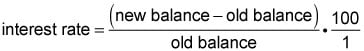Plug in the given values to find the rate: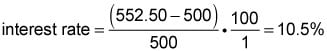This value is for three years of interest, so dividing by 3 gives you the annual simple interest rate, which equals 3.5%. Hence, Choice (A) is correct.